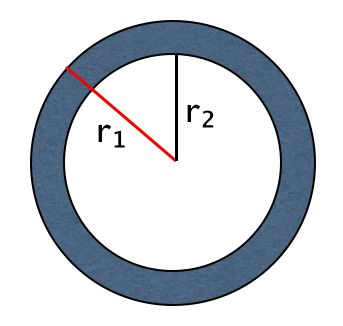Learn the 4 Things You Must Know Before Taking the GMAT!   Download it Now!

# Annulus

In the world of GMAT geometry, the more advanced problems combine geometric figures. You may know the rules of triangles or quadrilaterals or circles individually, but what if a couple of figures appear combined in the same question? Often those questions come in the form of finding a shaded area, and a particular type of shaded region question on the GMAT is called an annulus question.

The annulus is simply the region between concentric circles, as seen here:Now, the good news is that you won’t have to know the term annulus on the GMAT! It may be worth knowing at a cocktail party because it’s a funny word to say, but it’s not the type of obscure math term you need to fill your brain with on the GMAT. What you do need to know, however, is how to find the area of the annulus — i.e., how to find the area of the shaded region between two concentric circles.

There are really only two things you need to know to solve annulus questions:

1) The area formula for a circle:

Area of a circle = πr²

2) The area of the annulus is calculated by subtracting the smaller area’s circle from the larger circle’s area. (Note: This is essentially the way you calculate the area of any shaded region on the GMAT). Thus,

Area of annulus = πr₁² – πr₂²

So, if the radius of the larger circle is 6 and the area of the smaller circle is 4, then the area of the annulus is π(6)² – π(4)² = 36π – 16π = 20π.

Give this example a try:

Q1: A path 4 feet wide surrounds a circular lawn whose diameter is 20 feet. What is the area of the path?

To answer this — or any — annulus problem, make sure you’re clear on r₁ and r₂. In this question, we’re given the diameter of the smaller circle, so the radius is half of that, or 10ft. To be consistent with the figure above, we’ll call that r₂. Since the width of the path is 4 feet wide, then the radius of the larger circle (r₁) is simply 10 + 4 = 14ft.

Now you simply plug r₁ and r₂ into the annulus area formula to find the answer:

Area of the path (annulus) = π(14)² – π(10)² = 196π – 100π = 96π.

Congratulations if you got that!

Now, here’s a variation of the same question. See how you do!

Q2: If the area of a circular lawn is 64π and it is surrounded by a path that has an area of 36π, then what is the width of the path?